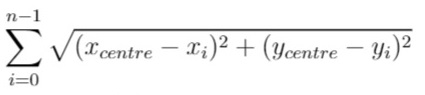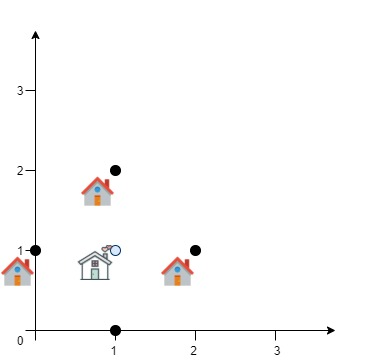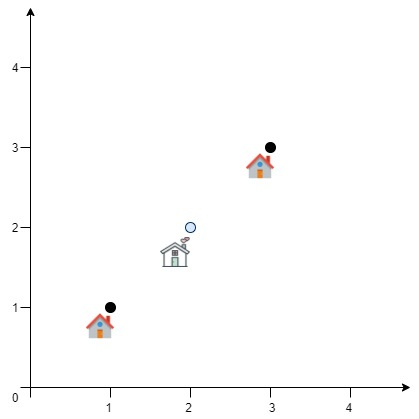1515. Best Position for a Service Centre
Hard
207
247

A delivery company wants to build a new service center in a new city. The company knows the positions of all the customers in this city on a 2D-Map and wants to build the new center in a position such that the sum of the euclidean distances to all customers is minimum.

Given an array `positions` where `positions[i] = [xi, yi]` is the position of the `ith` customer on the map, return the minimum sum of the euclidean distances to all customers.

In other words, you need to choose the position of the service center `[xcentre, ycentre]` such that the following formula is minimized:Answers within `10-5` of the actual value will be accepted.

Example 1:```Input: positions = [[0,1],[1,0],[1,2],[2,1]]
Output: 4.00000
Explanation: As shown, you can see that choosing [xcentre, ycentre] = [1, 1] will make the distance to each customer = 1, the sum of all distances is 4 which is the minimum possible we can achieve.
```

Example 2:```Input: positions = [[1,1],[3,3]]
Output: 2.82843
Explanation: The minimum possible sum of distances = sqrt(2) + sqrt(2) = 2.82843
```

Constraints:

• `1 <= positions.length <= 50`
• `positions[i].length == 2`
• `0 <= xi, yi <= 100`
Accepted
12.3K
Submissions
33K
Acceptance Rate
37.3%

Seen this question in a real interview before?
1/4
Yes
No

Discussion (0)

Related Topics